﻿ Equation Options

# Equation Options

Top  Previous  Next

# Accessing Equation Options

There are many options for the rendering and interpretation of equations.  These are accessed with this button.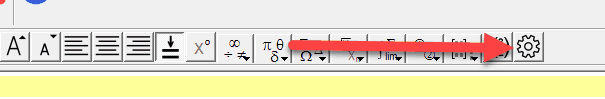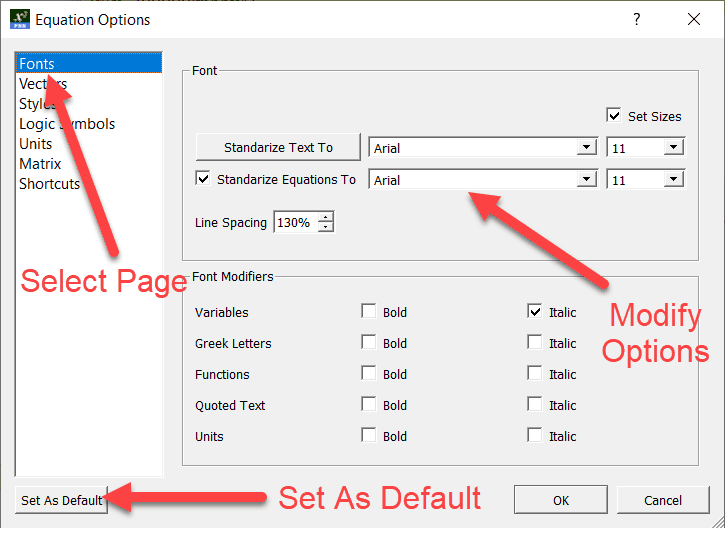The options are discussed later in the manual.

# Standardizing Equations

If you choose to use the current text font, your equation will just use the font that is currently selected in the text box.  This means that if you are typing in Arial 12pt, your equation will also be in Arial 12pt.

Many users want to standardize on one font for equations (often Times New Roman) regardless of the text around the equation.  You can choose this by choosing Standardize Equations and selecting the font you want to use. This font, and optionally size, will be used for equations regardless of any other formatting in your equation.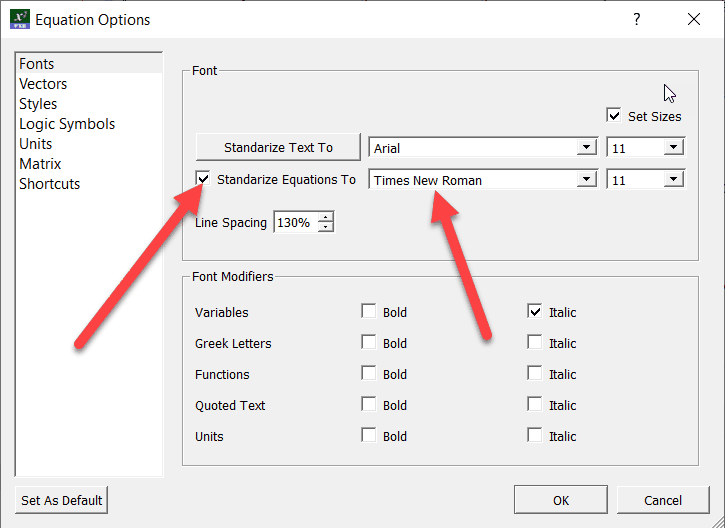Some users want to standardize even further and always use a specific font size for equations - regardless of the text around the equation.  You can do this by checking the Set Sizes option.

# Font Modifiers

Each component of an equation can be formatted differently.  You can format functions in bold, italicise variables and lots of other options - all automatically.  Just set the options for each of the different parts of your equation.  Most users choose to italicise variables.

Automatic font modifiers you choose can be overridden by selecting parts of the equation and manually changing the formatting.

# Column Vectors

If you wish to enter vectors in the vertical format, they are simply entered as (3,2) using a comma as a separator.  Three-dimensional vectors are entered in the same way.  If you normally use a comma as a decimal point, the equation tool will automatically detect this and you must use a semicolon to separate components of a vertical vector.

Examples: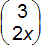is entered as (3,2x) or (3;2x)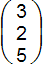is entered as (3,2,5) or (3;2;5)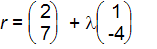is entered as r=(2,7)+la(1,-4) or r=(2;7)+la(1;-4)

You must have selected the Use Commas for Column Vectors option to allow FX Equation to detect column vectors and matrices.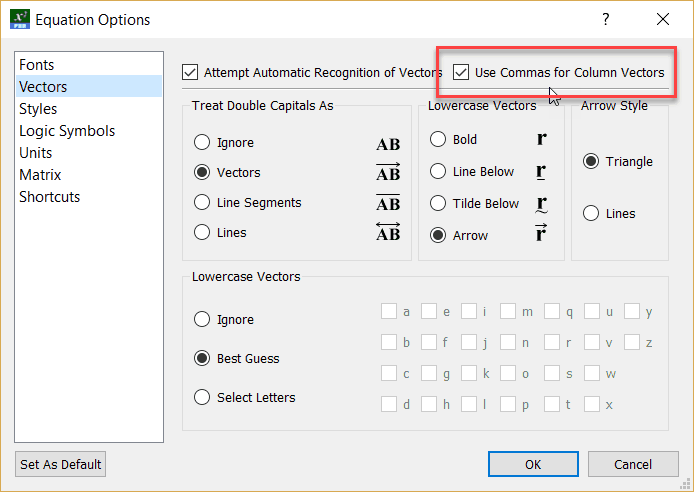You can also control the use of commas for column vectors on the main toolbar.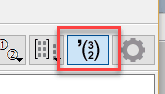# Automatic Detection of Vectors

The equation tool can automatically recognize some vectors if you choose to turn the feature on.  The equation tool can recognize some lowercase vectors and double capital vectors.  This feature is activated by default and can be controlled by on the Equation / Vectors option screen.

# Lowercase Vectors

The equation tool will only detect lowercase vectors if automatic recognition is switched on.

If you are using vectors of the form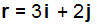The equation tool can automatically recognise that you are typing a vector and make the r, i and j bold. The equation tool can also be set to indicate vectors using lines, tildes or arrows, depending on your preference.

This automatic recognition of vectors can save you hours of time, but the equation tool may sometimes get the automatic recognition incorrect.  By default, the equation tool will take its "best guess", you can also turn this feature off or alternatively, explicitly tell the equation tool which variables to recognise as vectors.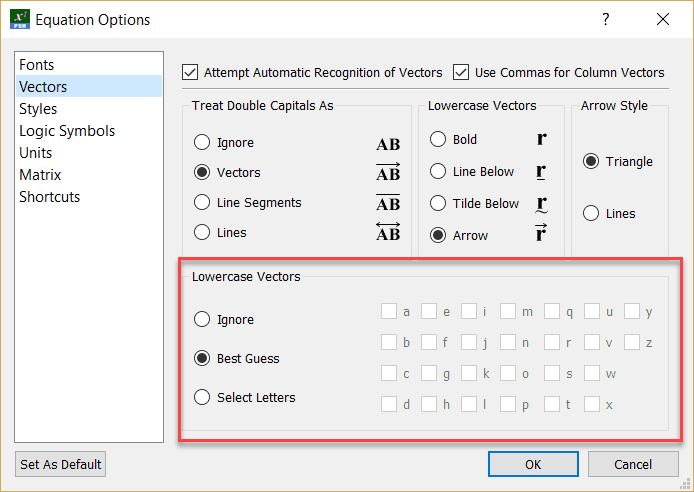It is important to remember that you do not need to tell the equation tool that you are working with a vector, the equation tool will automatically identify this.

## Example: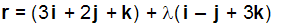is entered as r=(3i+2j+k)+la(i-j+3k)

Lowercase vectors can be display bold, with an arrow over the top or with a line or tilde underneath the vector.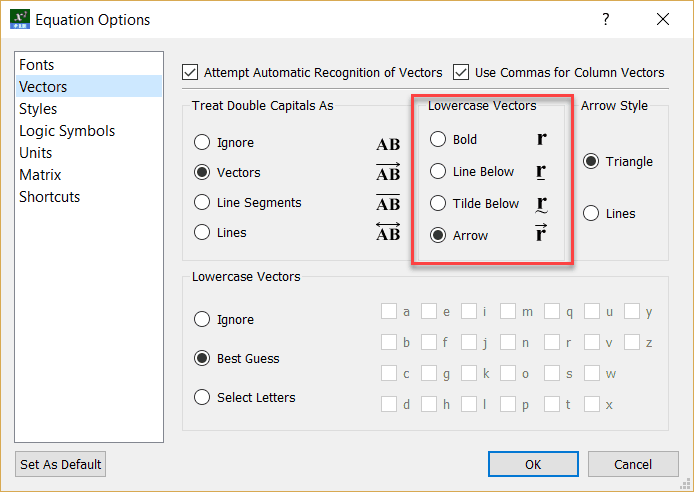# Double Capitals

The equation tool will only detect Double Capital vectors if automatic recognition is switched on.

Double capital letters are used to represent line segments, rays and vectors.  The equation tool can automatically place an annotation over double capitals.  You would use this feature if you were, for example, entering a lot of position vectors in an exam.

Examples: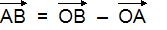is entered as AB = OB - OA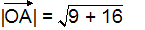is entered as |OA|=sr(9+16)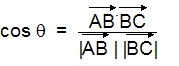is entered as cos th = (AB.BC)/(|AB | |BC|)

The annotation used by the equation tool can be set using the Vector Options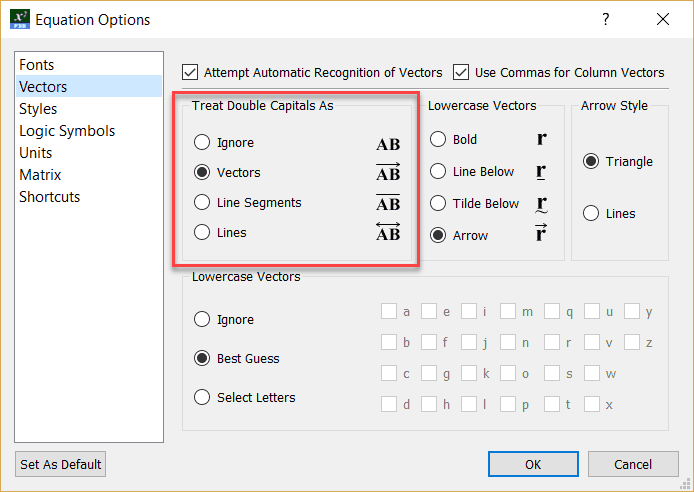#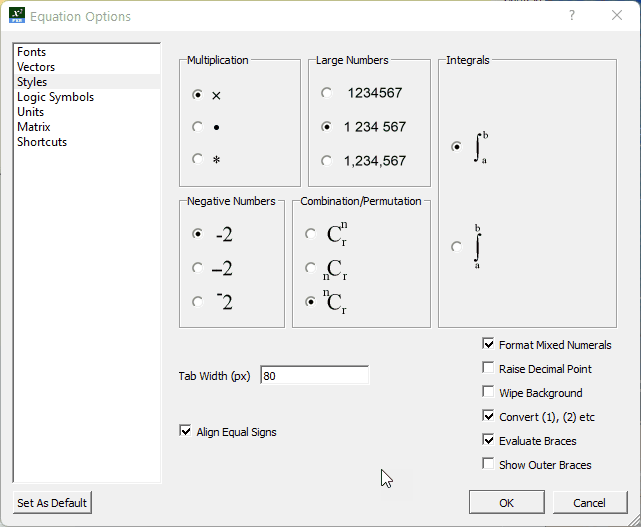## Multiplication Sign

When using the equation tool, you enter a multiplication sign using the * key.  The equation tool can display your sign in one of three ways, as an ´, a raised dot ( × ) or an *.

The equation tool will display any entered multiplication sign AND the multiplication sign in scientific notation using this setting.

## Large Numbers

The equation tool can automatically format large numbers using spaces or commas between the thousand groups.

## Raising the Decimal Point

Some locations prefer that the decimal point is raised.

ie.        3·2                rather than                3.2

This option will only take effect if you use a dot as a decimal point.

## Integrals

Integrals can be drawn in two different ways - with the bounds to the right of the integral sign or with the bounds above and below.

## Mixed Numerals

Mixed Numerals are created automatically if you have selected the Format Mixed Numerals option.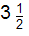is entered as 3 1/2

## Negative Numbers

The equation tool offers you the option to display negative numbers differently to subtraction signs.

The three options produce these results.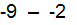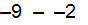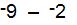# Evaluating Braces

The styles page allows you to turn on evaluation of braces. This is discussed in more detail in The Inline Calculator

# Logic Symbols

The equation tool can use a selection of different symbols for logic statements.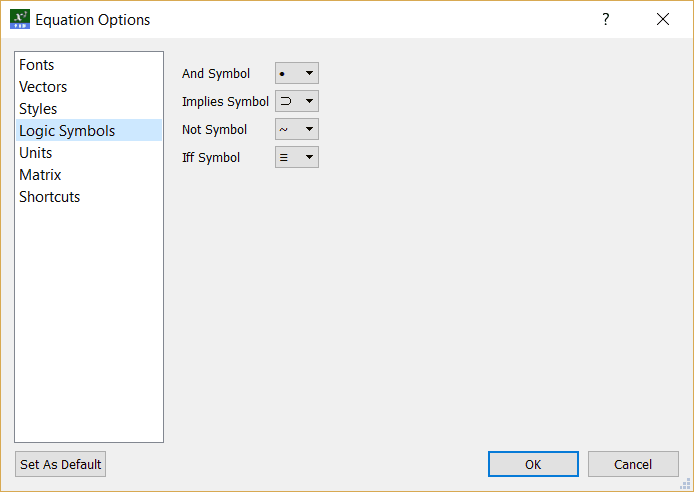# Units

The equation tool can detect units and display them using different settings.  This allows you to clearly delineate things such as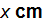All you need to do to use this feature is to tell the equation tool what to consider a unit.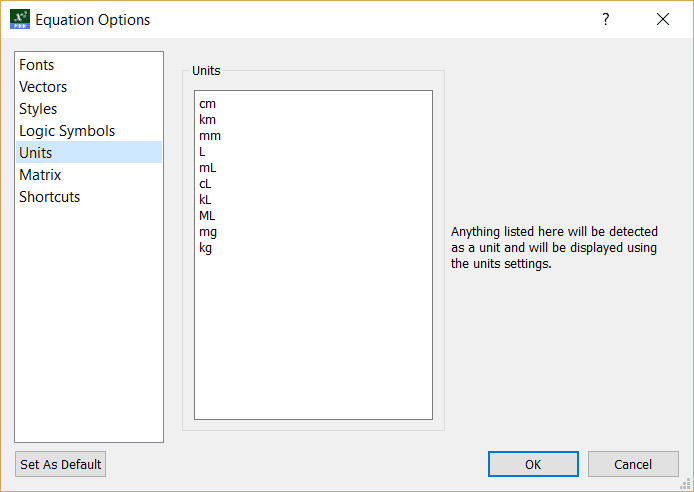These units are our suggestions.  You can set the units to whatever you require - and change them on an equation by equation basis.

Single letter, lower case units (eg s, g, h, and m) are usually best left off this list.  Putting them on the list causes FX Equation to behave counter-intuitively, although totally logically.

For example...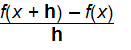The h has been formatted using the units formatting but not the way the user was expecting.

# Matrix

You can adjust vertical and horizontal spacing for your matrices as well as alignment.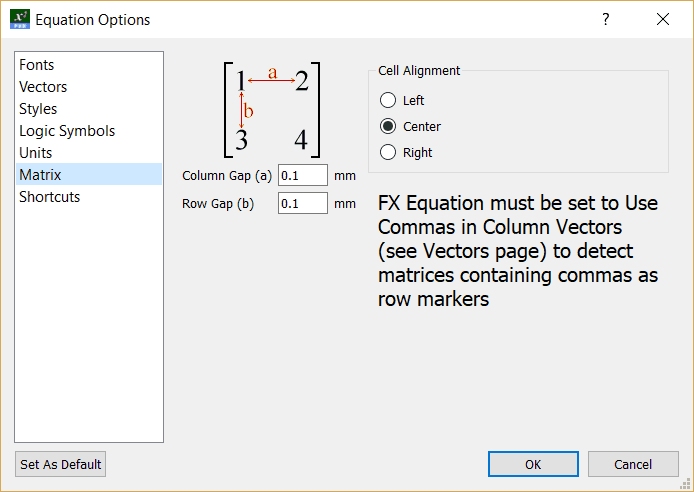# Shortcuts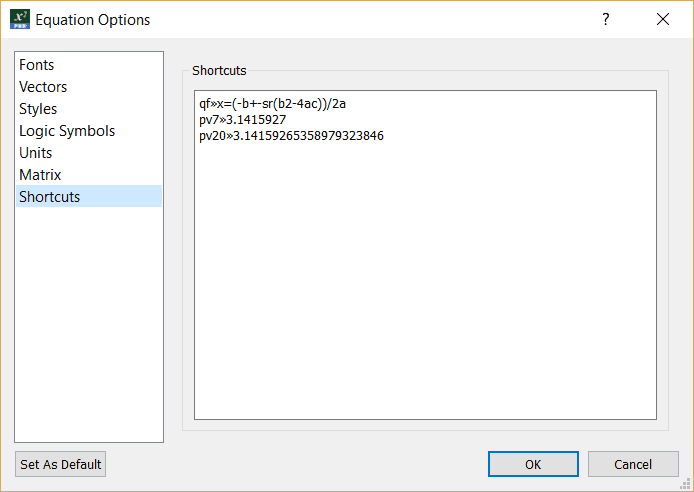Each shortcut is on a separate line.  To edit a shortcut, just move the cursor into the shortcut and start typing.  To create a new shortcut, move the cursor to the bottom of the list and start typing.

Shortcuts are formatted as

The code - followed by the tab key - followed by the desired output

## qf»x=(-b+-sr(b2-4ac))/2a

The qf is the code

The » is produced by pushing the tab key

The x=(-b+-sr(b2-4ac))/2a  is the desired output.